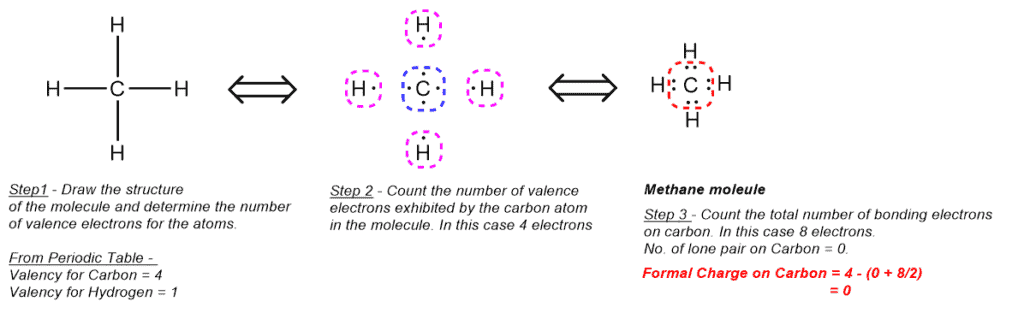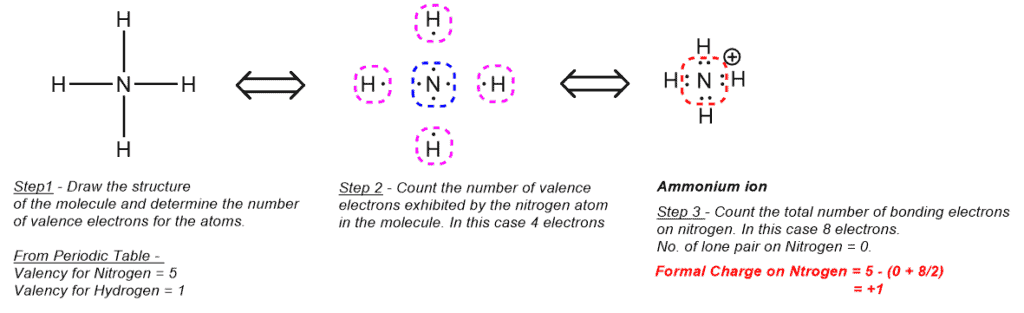# Formal Charge

## Formal Charge Definition:

A formal charge is a hypothetical charge associated with any atom when all bonding electrons are shared equally among the atoms. Hence, to say that an atom has a formal charge means that the atom does not exhibit the appropriate number of valence electrons.

## Formal Charge Explained:

The best way to identify a formal charge on an atom is by drawing its Lewis dot structure that helps us identify the total number of valence electrons of the atom (bonding electrons + non-bonding electrons). Once we know the accurate number of valence electrons, we may now calculate the formal charge using the formula below and determine whether or not the atom exhibit the appropriate number of valence electrons. If it does not, then we get a non-zero value for the formal charge.### Examples –

• Formal Charge on Carbon in Methane• Formal charge on Nitrogen in Ammonium ion×

Cart